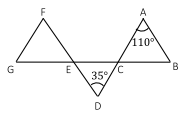# Free GMAT Test Questions

Question 1 of 1
ID: GMAT-PS-7
Section: Quantitative Reasoning - Problem Solving
Topics: Geometry; Triangles
Difficulty level: Medium

(Practice Mode: Single selected Question » Back to Overview)

If in the figure below, triangles ABC and FGE are isosceles, what is the measure of $\angle$FGE? (Figure: Not to scale)A$15^\circ$
B$35^\circ$
C$45^\circ$
D$75^\circ$
E$110^\circ$